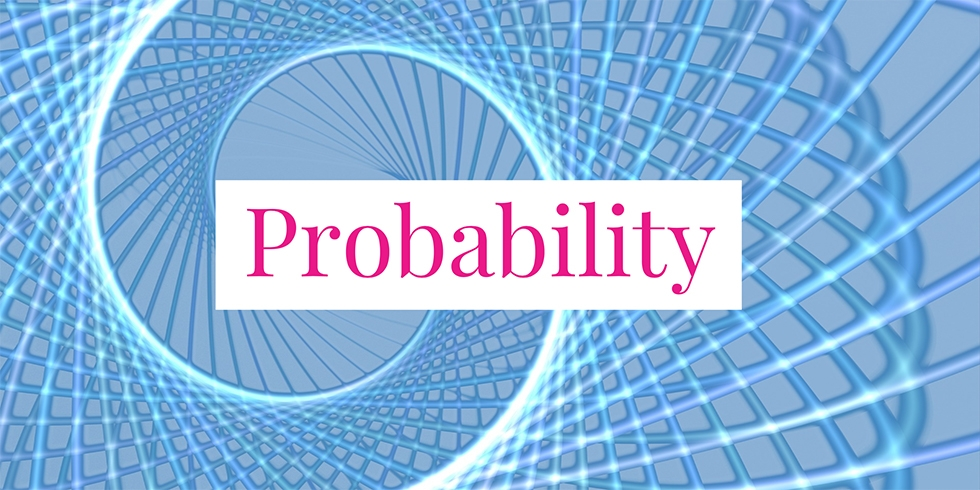# Linearity of Expectations E(X+Y) = E(X) + E(Y)## Problem 743

Let $X, Y$ be discrete random variables. Prove the linearity of expectations described as
$E(X+Y) = E(X) + E(Y).$Add to solve later

## Solution.

The joint probability mass function of the discrete random variables $X$ and $Y$ is defined by
$p(x, y) = P(X=x, Y=y).$ Note that the probability mass function of $X$ can be obtained from $p(x, y)$ by
\begin{align*}
p_X(x) &= P(X=x)\\
& = \sum_{y} p(x, y).
\end{align*}
Similarly, the probability mass function of $Y$ is expressed as
$p_Y(y) = \sum_{x} p(x, y).$

Using these equalities and the definition of expectations, we can compute $E(X+Y)$ as follows.

\begin{align*}
E(X+Y) &= \sum_{x} \sum_{y} (x+y) p(x, y)\\
&= \sum_{x} \sum_{y}\left(x p(x, y) + y p(x, y)\right)\\
&= \sum_{x} \sum_{y} x p(x, y) + \sum_{x} \sum_{y} y p(x, y)\\
&= \sum_x x \sum_y p(x,y) + \sum_y y \sum_x p(x,y)\\
&= \sum x p_X(x) + \sum_y y p_Y(y)\\
&= E(X) + E(Y)
\end{align*}

This proves the desired linearity of expectations $E(X+Y) = E(X) +E(Y)$.Add to solve later

### More from my site

#### You may also like...

This site uses Akismet to reduce spam. Learn how your comment data is processed.

###### More in Probability##### Successful Probability of a Communication Network Diagram

Consider the network diagram in the figure. The diagram consists of five links and each of them fails to communicate...

Close